# Introduction to theft

#### 2022-05-31

library(theft)

## Core calculation functions

We are going to use a dataset that comes standard with theft called simData. This dataset contains a collection of randomly generated time series for six different types of processes. The dataset can be accessed via:

theft::simData

The data follows the following structure:

head(simData)
#>        values timepoint               id        process
#> 1: -0.6264538         1 Gaussian Noise_1 Gaussian Noise
#> 2:  0.1836433         2 Gaussian Noise_1 Gaussian Noise
#> 3: -0.8356286         3 Gaussian Noise_1 Gaussian Noise
#> 4:  1.5952808         4 Gaussian Noise_1 Gaussian Noise
#> 5:  0.3295078         5 Gaussian Noise_1 Gaussian Noise
#> 6: -0.8204684         6 Gaussian Noise_1 Gaussian Noise

### Calculating feature summary statistics

The core function that automates the calculation of the feature statistics at once is calculate_features. You can choose which subset of features to calculate with the feature_set argument. The choices are currently "catch22", "feasts", "Kats", "tsfeatures", "tsfresh", and/or "TSFEL".

Note that Kats, tsfresh and TSFEL are Python packages. The R package reticulate is used to call Python code that uses these packages and applies it within the broader tidy data philosophy embodied by theft. At present, depending on the input time-series, theft provides access to ~1200 features. Prior to using theft (only if you want to use the Kats, tsfresh or TSFEL feature sets - the R-based sets will run fine) you should have a working Python installation and download Kats using the instructions located here, tsfresh here or TSFEL here.

Here is an example with the catch22 set:

feature_matrix <- calculate_features(data = simData,
id_var = "id",
time_var = "timepoint",
values_var = "values",
group_var = "process",
feature_set = "catch22",
seed = 123)

Note that for the catch22 set you can set the additional catch24 argument to calculate the mean and standard deviation in addition to the standard 22 features:

feature_matrix <- calculate_features(data = simData,
id_var = "id",
time_var = "timepoint",
values_var = "values",
group_var = "process",
feature_set = "catch22",
catch24 = TRUE,
seed = 123)

Note that if you want to use any of the Python-based packages, you must first tell R (prior to running calculate_features) which Python location on your computer contains the installed libraries. This can be done via the init_theft function, where path_to_python is a string specifying the filepath location. For example, for me (Trent), the filepath to the correct Python is "~/opt/anaconda3/bin/python" which is what I would enter for the path_to_python argument. You only need to call init_theft once per R session.

init_theft(path_to_python)

A tidy dataframe of most of the included features and the set they correspond to is available in the dataframe feature_list:

head(feature_list)
#>   feature_set                  feature
#> 1     catch22       DN_HistogramMode_5
#> 2     catch22      DN_HistogramMode_10
#> 3     catch22                CO_f1ecac
#> 4     catch22           CO_FirstMin_ac
#> 5     catch22 CO_HistogramAMI_even_2_5
#> 6     catch22            CO_trev_1_num

NOTE: If using the tsfresh feature set, you might want to consider the tsfresh_cleanup argument to calculate_features. This argument defaults to FALSE and specifies whether to use the in-built tsfresh relevant feature filter or not.

For a detailed comparison of the six feature sets, see this paper for a detailed review1.

## Data quality checks

The package comes with a function to visualise the data types of the calculated feature vectors. This is useful for inspecting which features might need to be dropped due to large proportions of undesirable (e.g., NA, NaN etc.) values.

plot_quality_matrix(feature_matrix)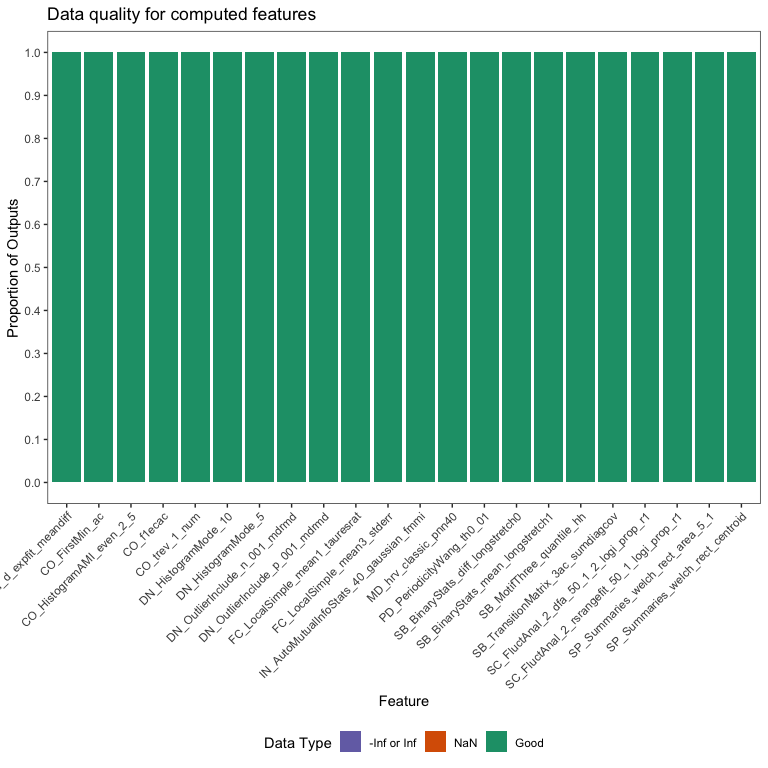## Normalising/scaling functions

Putting calculated feature vectors on an equal scale is crucial for any statistical or machine learning model as variables with high variance can adversely impact the model’s capacity to fit the data appropriately, learn appropriate weight values, or minimise a loss function. theft includes the functions normalise_feature_vector to rescale a vector of values (e.g. vector of values for all participants in a study on the SB_BinaryStats_mean_longstretch1 feature), and normalise_feature_frame to rescale a vector within a dataframe into a variety of different ranges for ease-of-use. Current transformations available in the package include:

• z-score - "z-score"
• Sigmoid - "Sigmoid"
• Outlier-robust Sigmoid (credit to Ben Fulcher for creating the original MATLAB version) - "RobustSigmoid"
• Min-max - "MinMax"

Dataframe-based normalisation can be performed using the following:

normed <- normalise_feature_frame(feature_matrix,
names_var = "names",
values_var = "values",
method = "RobustSigmoid")

## Data visualisation and low-dimensional project functions

The package also comes with a built-in plotting engine for calculating and visualising many types of statistical graphics:

• Feature by time-series matrix as a heatmap
• Low dimensional projections of the feature space as a scatterplot (along with numerical results)
• Pairwise correlation matrix as a heatmap

### Feature matrices

The function plot_all_features (deprecated version: plot_feature_matrix) takes the calculated features and produces a ggplot object heatmap showing the feature vectors across the x axis and each time series down the y axis. Prior to plotting, the function hierarchically clusters the data across both rows and columns to visually highlight the empirical structure. Note that you have several options for the hierarchical clustering linkage algorithm to use:

• "average" (default)
• "ward.D"
• "ward.D2"
• "single"
• "complete"
• "mcquitty"
• "median"
• "centroid"

See the hclust documentation for more information.

Note that the legend for this plot (and other matrix visualisations in theft) have been discretised for visual clarity as continuous legends can be difficult to interpret meaningful value differences easily.

plot_all_features(feature_matrix,
is_normalised = FALSE,
id_var = "id",
method = "RobustSigmoid",
clust_method = "average",
interactive = FALSE)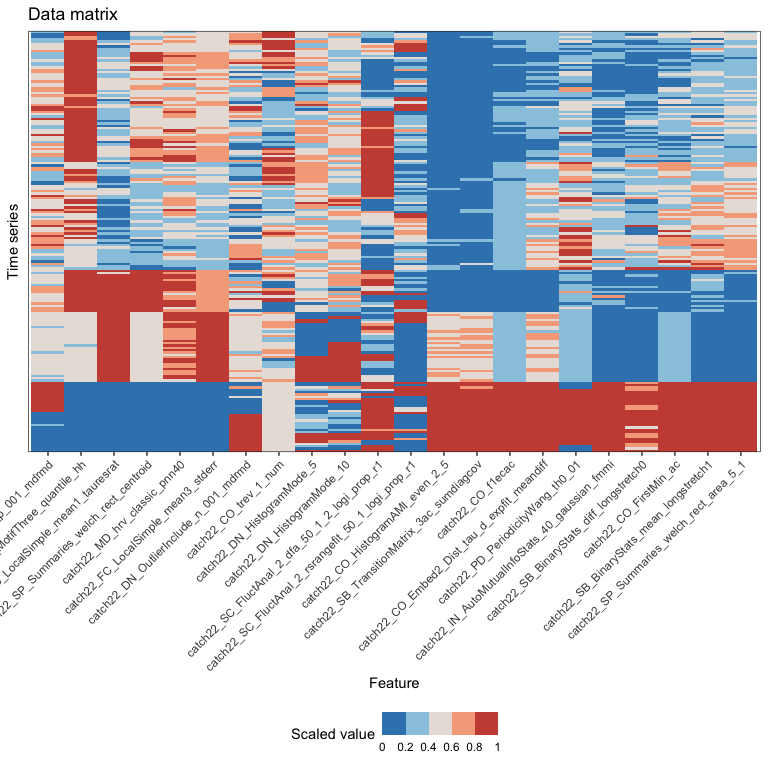If you set interactive = TRUE the function will return an interactive plot using plotly which lets you hover over entries in the matrix to better understand which is which. This is especially useful if your dataset is large.

plot_feature_matrix(feature_matrix,
is_normalised = FALSE,
id_var = "id",
method = "RobustSigmoid",
clust_method = "average",
interactive = TRUE)

### Low dimensional projections

The function plot_low_dimension takes the calculated features, calculates a principal components analysis (PCA) or t-distributed stochastic neighbour embedding (t-SNE), and produces a ggplot object scatterplot showing the first and second principal components on the x and y axis respectively, their individual explained variance (if PCA is selected), and each time series as a point. If plot = FALSE the function returns a dataframe of results. If a variable is specified in the group_var argument, the scatterplot points will be automatically coloured by group.

plot_low_dimension(feature_matrix,
is_normalised = FALSE,
id_var = "id",
group_var = "group",
method = "z-score",
low_dim_method = "PCA",
plot = TRUE,
show_covariance = TRUE,
seed = 123)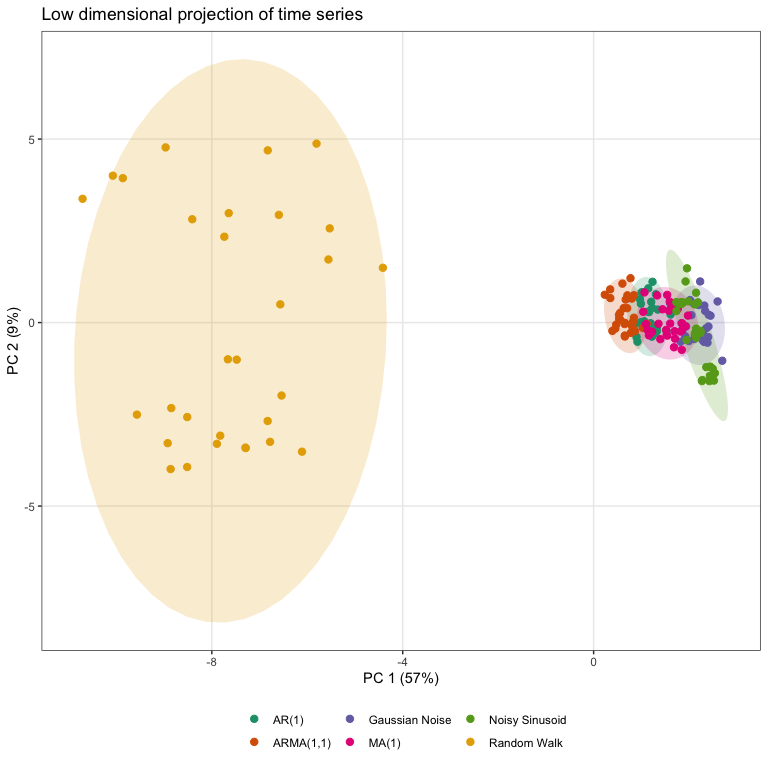Alternatively, a t-SNE version can be specified in a similar fashion, with the perplexity hyperparameter able to be controlled by the user. Typical values for this range between 5 and 50, depending on the size of the data. At lower levels of perplexity, local variations tend to dominate, while at very high levels of perplexity, results can be uninterpretable as clusters can merge. See this interactive article for a detailed review.

plot_low_dimension(feature_matrix,
is_normalised = FALSE,
id_var = "id",
group_var = "group",
method = "z-score",
low_dim_method = "t-SNE",
perplexity = 10,
plot = TRUE,
show_covariance = FALSE,
seed = 123)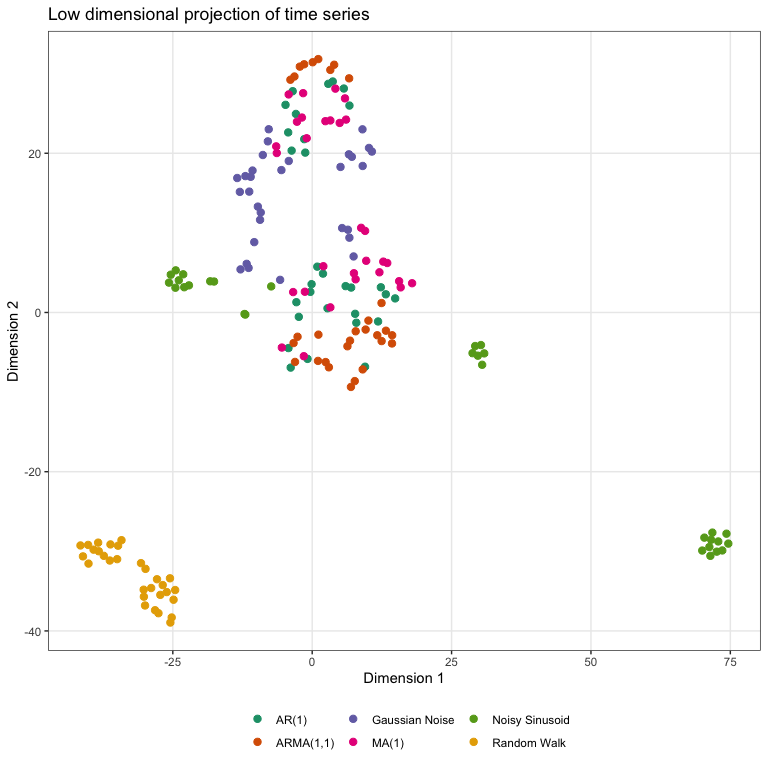### Pairwise correlations

#### Time-series correlations

The function plot_ts_correlations automates the calculation of correlations between values of each unique time series, the hierarchical clustering of rows and columns of these correlations, and the production of a final heatmap graphic. You can switch the default Pearson correlation to a Spearman rank correlation by changing the cor_method argument to spearman. This visualisation might not always be highly informative, but it can be useful to take a quick glance regardless.

plot_ts_correlations(simData,
is_normalised = FALSE,
id_var = "id",
time_var = "timepoint",
values_var = "values",
method = "RobustSigmoid",
cor_method = "pearson",
clust_method = "average",
interactive = FALSE)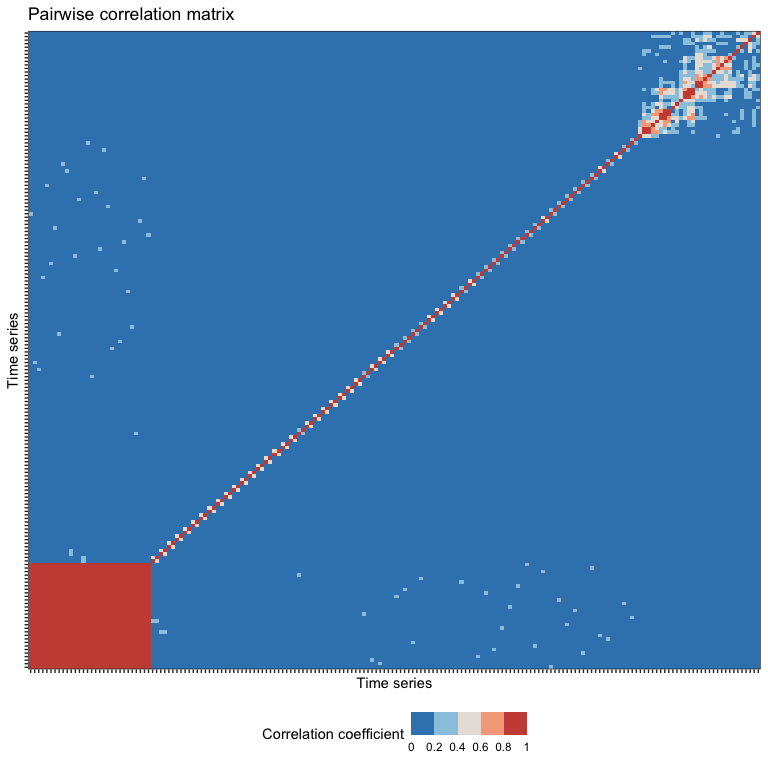Again, if you set interactive = TRUE the function will return an interactive plot using plotly which lets you hover over entries in the matrix to better understand which is which.

plot_ts_correlations(simData,
is_normalised = FALSE,
id_var = "id",
time_var = "timepoint",
values_var = "values",
method = "RobustSigmoid",
cor_method = "spearman",
clust_method = "average",
interactive = TRUE)

#### Feature vector correlations

Similarly, one can also plot correlations between feature vectors using plot_feature_correlations. The general argument structure is the same as plot_ts_correlations.

plot_feature_correlations(feature_matrix,
is_normalised = FALSE,
id_var = "id",
names_var = "names",
values_var = "values",
method = "RobustSigmoid",
cor_method = "pearson",
clust_method = "average",
interactive = FALSE)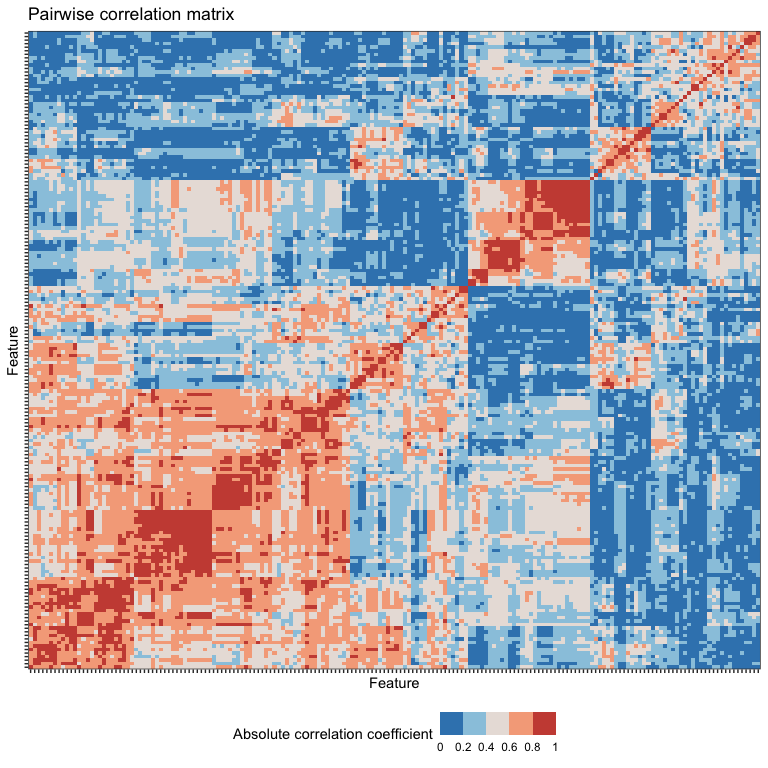## Time-series classification

### Feature-by-feature

Since feature-based time-series analysis has shown particular promise for classification problems, theft includes functionality for exploring group separation in addition to the low dimensional representation. The compute_top_features function takes the computed features and performs the following:

1. Processes the data ready for analysis
2. Computes a classification algorithm or statistical model on the entire input dataframe
3. Extracts individual feature performance from the classification algorithm using a designated performance metric
4. Filters the feature data to the top n performing features (where n is specified by the user)
5. Plots a feature $$\times$$ feature correlation matrix as a heatmap for the top performing features
6. Plots a group of violin plots coloured by class label for the top performing features

This analysis is useful because it can help guide researchers toward more efficient and appropriate interpretation of high-performing features (if any exist) and helps protect against over-interpretation of feature values.

This function returns an object with three components to summarise the above steps:

1. ResultsTable – a dataframe containing feature names, feature set membership, and performance statistics for the top performing features
2. FeatureFeatureCorrelationPlot – a ggplot containing the pairwise correlations between top performing features represented as a heatmap
3. ViolinPlots – a ggplot containing a matrix of violin plots showing class discrimination by feature

The code below produces analysis for a multiclass problem using a Gaussian process classifier with a radial basis function kernel. It implements a custom “empirical null” procedure for estimating a p-value based on classification accuracy of the real data compared to a distribution of null samples built from classification accuracies of the same data but with random class label shuffles. This is also known as permutation testing (see this document2 for a good overview). Note that higher numbers of permutation samples means that a better empirical null distribution can be generated. It is recommended to run 100-1000 permutations, though this comes with considerable computation time. We’ll enable a k-fold cross-validation procedure too for good measure. Note that using these procedures increases the computation time. Electing to not use an empirical null will be faster, but top features will be determined based off classification accuracy of features and not p-values. Further, when use_k_fold = FALSE, the model will instead be fit on all data and predict classes based off the same data (in caret language, this is equivalent to caret::trainControl(method = "none") and then calling predict on the input data and getting accuracy from the confusion matrix between the two). This will very likely lead to overfitting, but will return results orders of magnitude faster than if use_k_fold = TRUE.

Importantly, the argument null_testing_method has two choices:

1. model free shuffles – Generates num_permutations number of random shuffles of group labels and computes the proportion that match, returning a distribution of “accuracies”. This model is extremely fast as it involves no null model fitting
2. null model fits – Generates num_permutations number of models that are trained and tested on data where the class label is shuffled. This method is slow but fits separate null models for each num_permutations shuffle

We we only use model free shuffles throughout this tutorial to reduce computation time.

p_value_method also has two choices:

1. empirical – calculates the proportion of null classification accuracies that are equal to or greater than the main model accuracy
2. gaussian – calculates mean and standard deviation of the null distribution to analytically calculate p-value for main model against. Initial package testing indicated that the null distributions (especially for increasing num_permutations) were approximately Gaussian, meaning this approach can be feasibly used

Note that model free shuffles is incompatible with pool_empirical_null = TRUE as the null distributions would be exactly the same. Further, if you set pool_empirical_null = TRUE this will compute statistical analysis for each feature against the entire pooled empirical null classification results of all features. Setting it to FALSE will compute a p-value for each feature against only its empirical null distribution.

IMPORTANT NOTE: theft currently does not label any results as “statistically significant”. If you intend to in your work, please adjust for multiple comparisons when considering numerous results against a threshold (e.g., $$\alpha = 0.05$$) and ensure any decisions you make are grounded in careful thought.

The seed argument allows you to specify a number to fix R’s random number generator for reproducible results. It defaults to 123 if nothing is provided.

outputs <- compute_top_features(feature_matrix,
id_var = "id",
group_var = "group",
num_features = 10,
normalise_violin_plots = FALSE,
method = "RobustSigmoid",
cor_method = "pearson",
clust_method = "average",
use_balanced_accuracy = FALSE,
use_k_fold = TRUE,
num_folds = 10,
use_empirical_null =  TRUE,
null_testing_method = "model free shuffles",
p_value_method = "gaussian",
num_permutations = 10,
pool_empirical_null = FALSE,
seed = 123)

Each component is named and can be accessed through regular use of the $ operator or through list indexing (both return the same object). Here’s the top few rows: head(outputs$ResultsTable)
#>                                        feature  accuracy p_value_accuracy
#> 1     catch22_sp_summaries_welch_rect_area_5_1 0.9500000    3.583919e-134
#> 2     catch22_sp_summaries_welch_rect_centroid 0.8722222    1.977506e-109
#> 3         catch22_fc_local_simple_mean3_stderr 0.8222222     7.091601e-95
#> 4           catch22_sb_motif_three_quantile_hh 0.7777778     7.962008e-83
#> 5 catch22_co_embed2_dist_tau_d_expfit_meandiff 0.6944444     1.761311e-62
#> 6            catch22_co_histogram_ami_even_2_5 0.6722222     1.499138e-57
#>   classifier_name               statistic_name
#> 1   gaussprRadial Mean classification accuracy
#> 2   gaussprRadial Mean classification accuracy
#> 3   gaussprRadial Mean classification accuracy
#> 4   gaussprRadial Mean classification accuracy
#> 5   gaussprRadial Mean classification accuracy
#> 6   gaussprRadial Mean classification accuracy

We could equivalently achieve the same with:

head(outputs[])

The feature-feature correlation plots are accessed as the second object:

print(outputs$FeatureFeatureCorrelationPlot)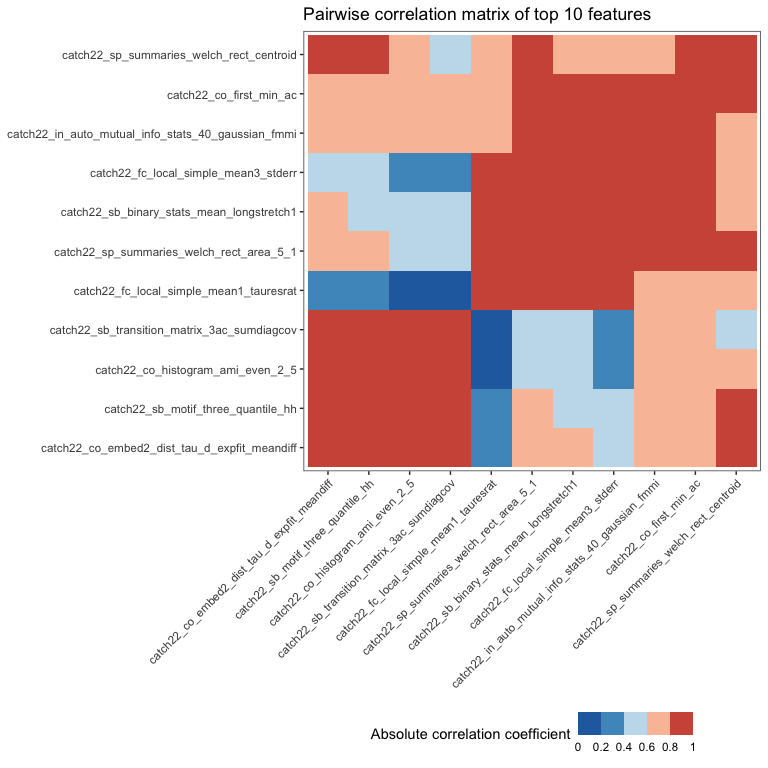The violin plots are accessed as the third object: print(outputs$ViolinPlots)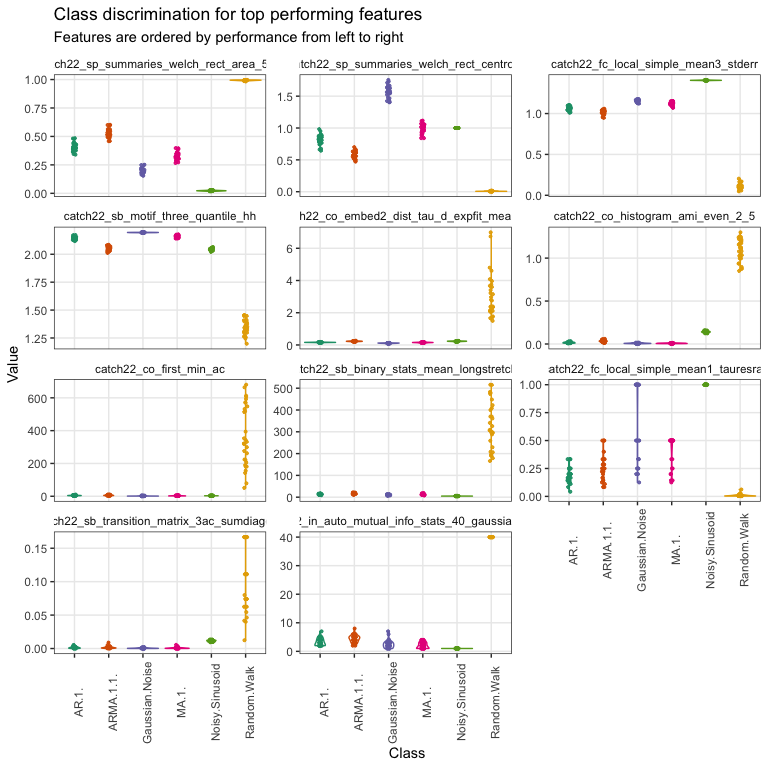For two-class problems, users can fit statistical models to directly compute p-values by specifying one of the following models as the string argument to test_method: "t-test", "wilcox" or "binomial logistic". theft has special procedures for these three options if a two-class problem is registered and thus none of the function arguments pertaining to empirical null testing or classification model parameters are used. For multiclass problems (and binary), users can specify any model name string that is a valid method name in the popular caret package and theft will pass this through to the relevant sub-procedures which will make use of the arguments relating to k-fold cross-validation, permutation testing, and p-value calculation.

### Multi-feature

A multi-feature option is also available. The fit_multivariable_classifier function fits all features at once instead of by individual features to estimate classification accuracy. This can be split by feature set (if the by_set argument is set to TRUE) to enable systematic comparisons between those made available in theft. To save computation time in this tutorial, we will only analyse catch22 (with by_set = TRUE to demonstrate automated plotting functionality), but comparing multiple calculated feature sets is recommended in practice.

Now we can use our multi-feature functionality (note the similarity in options to the single feature version we specified above):

multi_outputs <- fit_multi_feature_classifier(feature_matrix,
id_var = "id",
group_var = "group",
by_set = TRUE,
test_method = "svmLinear",
use_balanced_accuracy = TRUE,
use_k_fold = TRUE,
num_folds = 10,
use_empirical_null =  TRUE,
null_testing_method = "model free shuffles",
p_value_method = "gaussian",
num_permutations = 10,
seed = 123)

We can now access the various named objects returned by this function, which include:

1. FeatureSetResultsPlot (only if by_set is set to TRUE) – a ggplot displaying mean classification accuracy (or balanced classification accuracy if use_balanced_accuracy is set to TRUE) by feature set
2. TestStatistics – a dataframe containing a summary of test statistics (and p-values if use_empirical_null is set to TRUE)
3. RawClassificationResults – a dataframe containing classification accuracies results from each main and null prediction

Here’s the feature set comparison plot:

print(multi_outputs$FeatureSetResultsPlot)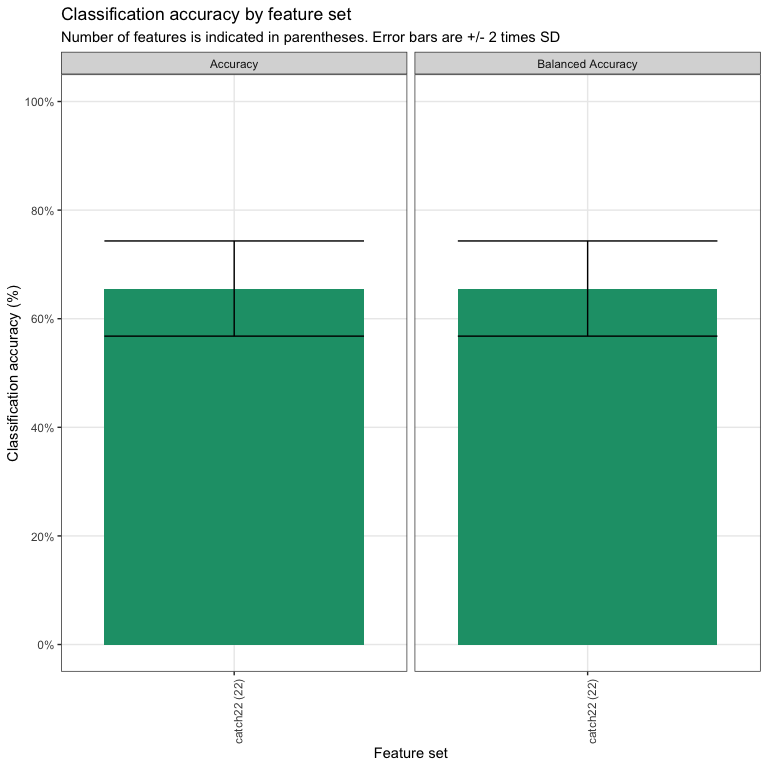Note that because we have balanced classes, in this case, accuracy $$=$$ balanced accuracy. Here’s the test statistics summary: head(multi_outputs$TestStatistics)
#>    method  accuracy p_value_accuracy balanced_accuracy
#> 1 catch22 0.6555556     5.452103e-54         0.6555556
#>   p_value_balanced_accuracy classifier_name
#> 1              5.452103e-54       svmLinear
#>                                                      statistic_name
#> 1 Mean classification accuracy and balanced classification accuracy

And here’s the raw classifier results:

head(multi_outputs\$RawClassificationResults)
#>    accuracy accuracy_sd balanced_accuracy balanced_accuracy_sd category
#> 1 0.6555556  0.04382281         0.6555556           0.04382281     Main
#> 2 0.1222222          NA         0.1222222                   NA     Null
#> 3 0.2277778          NA         0.2277778                   NA     Null
#> 4 0.1444444          NA         0.1444444                   NA     Null
#> 5 0.1444444          NA         0.1444444                   NA     Null
#> 6 0.1444444          NA         0.1444444                   NA     Null
#>                method num_features_used classifier_name
#> 1             catch22                22       svmLinear
#> 2 model free shuffles                NA       svmLinear
#> 3 model free shuffles                NA       svmLinear
#> 4 model free shuffles                NA       svmLinear
#> 5 model free shuffles                NA       svmLinear
#> 6 model free shuffles                NA       svmLinear
#>                                                      statistic_name
#> 1 Mean classification accuracy and balanced classification accuracy
#> 2 Mean classification accuracy and balanced classification accuracy
#> 3 Mean classification accuracy and balanced classification accuracy
#> 4 Mean classification accuracy and balanced classification accuracy
#> 5 Mean classification accuracy and balanced classification accuracy
#> 6 Mean classification accuracy and balanced classification accuracy

Note that for the multi-feature version, only valid caret method names are able to be used to specify a test_method.

## Reading and processing hctsa-formatted files

As theft is based on the foundations laid by hctsa, there is also functionality for reading in hctsa-formatted Matlab files and automatically processing them into tidy dataframes ready for feature extraction in theft. The process_hctsa_file function takes a string filepath to the Matlab file and does all the work for you, returning a dataframe with naming conventions consistent with other theft functionality. As per hctsa specifications for Input File Format 1, this file should have 3 variables with the following exact names: timeSeriesData, labels, and keywords. Here is an example using the Bonn University EEG dataset3.

d2 <- process_hctsa_file("https://cloudstor.aarnet.edu.au/plus/s/6sRD6IPMJyZLNlN/download")

1. T. Henderson and B. D. Fulcher, “An Empirical Evaluation of Time-Series Feature Sets,” 2021 International Conference on Data Mining Workshops (ICDMW), 2021, pp. 1032-1038, doi: 10.1109/ICDMW53433.2021.00134.↩︎

2. Rice, Ken & Lumley, Thomas. (2008) “Permutation tests”↩︎

3. Andrzejak, Ralph G., et al. (2001) “Indications of nonlinear deterministic and finite-dimensional structures in time series of brain electrical activity: Dependence on recording region and brain state.” Physical Review E 64(6): 061907↩︎